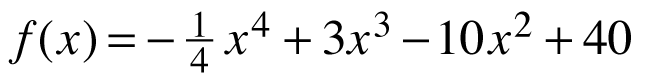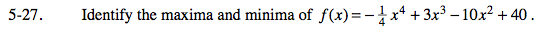### Home > CALC > Chapter 5 > Lesson 5.1.3 > Problem5-27

5-27.

Identify the maxima and minima of. Homework Help ✎Find CANDIDATES for maxima and minima by setting the first derivative equal to 0.

Decide if each candidate is a maximum, minimum or neither. You could check for slope change by evaluating the 1st-derivative at points close to each candidate. You could evaluate each candidate in the 2nd-derivative. If the graph is concave up, it cannot have a local max. And vice versa.

Recall that maxima and minima are y-values, not x-values. So use f(x) to find the y-value of each candidate.

(0, 40) is a local maximum, (4, 8) is a local minimum, (5, 8.75) is a local maximum, and (5, 8.75) is the global maximum. There is no global minimum.